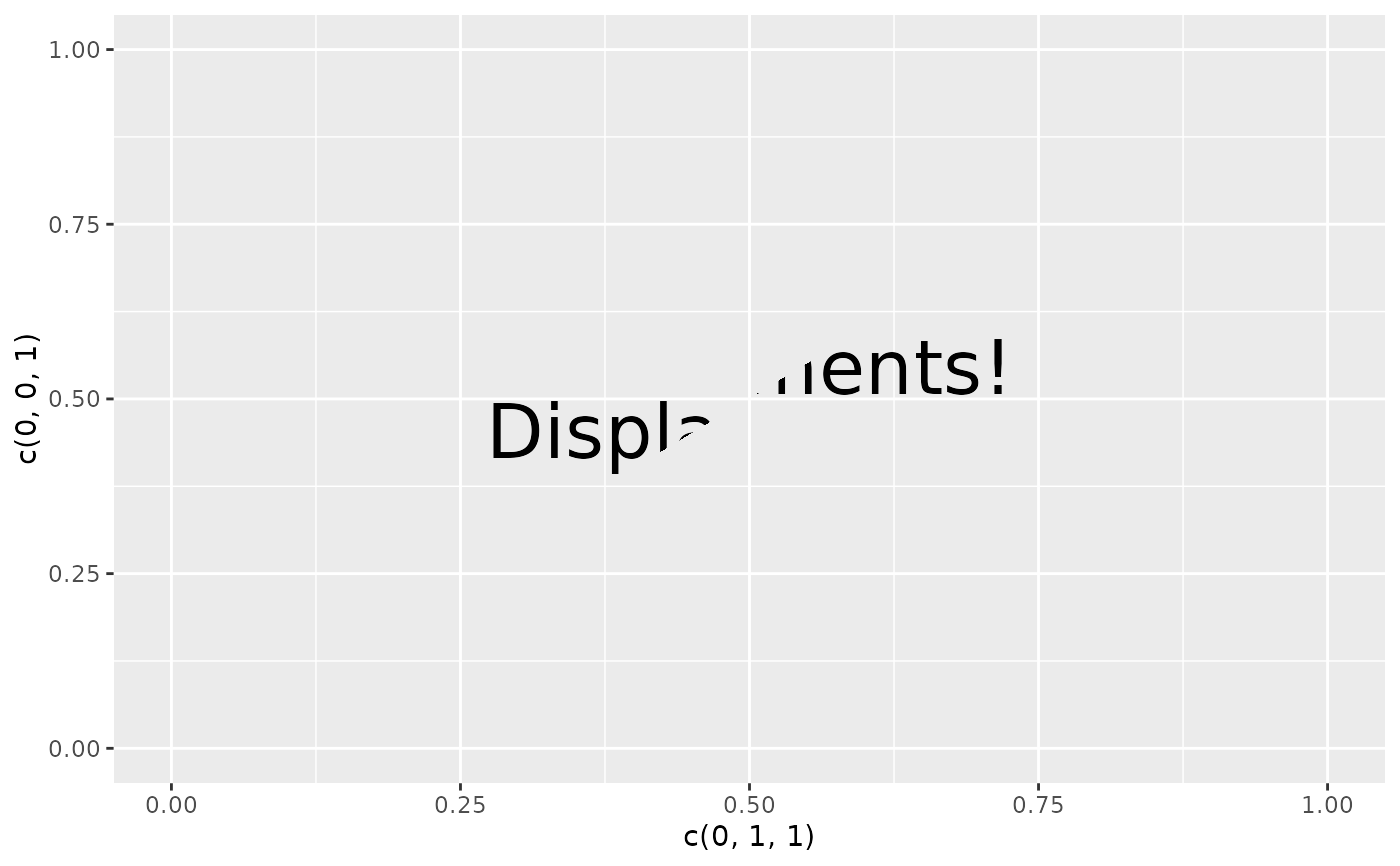This filter displaces the pixels based on the colour values of another layer or raster object. As such it can be used to distort the content of the layer.

with_displacement(x, x_map, y_map = x_map, x_scale = 1, y_scale = x_scale, ...)

## Arguments

x

A ggplot2 layer object, a ggplot, a grob, or a character string naming a filter

x_map, y_map

The displacement maps to use. Can either be a string identifying a registered filter, or a raster object. The maps will be resized to match the dimensions of x. Only one channel will be used - see the docs on channels for info on how to set them.

x_scale, y_scale

How much displacement should a maximal channel value correspond to? If a numeric it will be interpreted as pixel dimensions. If a unit object it will be converted to pixel dimension when rendered.

...

Arguments to be passed on to methods. See the documentation of supported object for a description of object specific arguments.

## Value

Depending on the input, either a grob, Layer, list of Layers, guide, or element object. Assume the output can be used in the same context as the input.

## Examples

library(ggplot2)
ggplot() +
as_reference(
geom_polygon(aes(c(0, 1, 1), c(0, 0, 1)), colour = NA, fill = 'magenta' ),
id = "displace_map"
) +
with_displacement(
geom_text(aes(0.5, 0.5, label = 'Displacements!'), size = 10),
x_map = ch_red("displace_map"),
y_map = ch_blue("displace_map"),
x_scale = unit(0.025, 'npc'),
y_scale = unit(0.025, 'npc')
)